• 2020-10-29 14:10:22

1.画最简单的直线图

代码如下：

import numpy as np

import matplotlib.pyplot as plt

x=[0,1]

y=[0,1]

plt.figure()

plt.plot(x,y)

plt.savefig("easyplot.jpg")

结果如下：代码解释：

#x轴，y轴

x=[0,1]

y=[0,1]

#创建绘图对象

plt.figure()

#在当前绘图对象进行绘图（两个参数是x,y轴的数据）

plt.plot(x,y)

#保存图象

plt.savefig("easyplot.jpg")

2.给图加上标签与标题

上面的图没有相应的X，Y轴标签说明与标题

在上述代码基础上，可以加上这些内容

代码如下：

import numpy as np

import matplotlib.pyplot as plt

x=[0,1]

y=[0,1]

plt.figure()

plt.plot(x,y)

plt.xlabel("time(s)")

plt.ylabel("value(m)")

plt.title("A simple plot")

结果如下：代码解释：

plt.xlabel("time(s)") #X轴标签

plt.ylabel("value(m)") #Y轴标签

plt.title("A simple plot") #标题

3.画sinx曲线

代码如下：

# -*- coding: utf-8 -*-

import numpy as np

import matplotlib.pyplot as plt

#设置x,y轴的数值（y=sinx）

x = np.linspace(0, 10, 1000)

y = np.sin(x)

#创建绘图对象，figsize参数可以指定绘图对象的宽度和高度，单位为英寸，一英寸=80px

plt.figure(figsize=(8,4))

#在当前绘图对象中画图（x轴,y轴,给所绘制的曲线的名字，画线颜色，画线宽度）

plt.plot(x,y,label="$sin(x)$",color="red",linewidth=2)

#X轴的文字

plt.xlabel("Time(s)")

#Y轴的文字

plt.ylabel("Volt")

#图表的标题

plt.title("PyPlot First Example")

#Y轴的范围

plt.ylim(-1.2,1.2)

#显示图示

plt.legend()

#显示图

plt.show()

#保存图

plt.savefig("sinx.jpg")

结果如下：4.画折线图

代码如下：

# -*- coding: utf-8 -*-

import numpy as np

import matplotlib.pyplot as plt

#X轴，Y轴数据

x = [0,1,2,3,4,5,6]

y = [0.3,0.4,2,5,3,4.5,4]

plt.figure(figsize=(8,4)) #创建绘图对象

plt.plot(x,y,"b--",linewidth=1) #在当前绘图对象绘图（X轴，Y轴，蓝色虚线，线宽度）

plt.xlabel("Time(s)") #X轴标签

plt.ylabel("Volt") #Y轴标签

plt.title("Line plot") #图标题

plt.show() #显示图

plt.savefig("line.jpg") #保存图

结果如下：总结

以上就是本文关于python绘制简单折线图代码示例的全部内容，希望对大家有所帮助。感兴趣的朋友可以继续参阅本站其他相关专题。如有不足之处，欢迎留言指出。感谢朋友们对本站的支持！

更多相关内容
• 主要介绍了python绘制简单折线图代码示例，具有一定借鉴价值，需要的朋友可以参考下。
• 今天小编就为大家分享一篇修改python plot折线图的坐标轴刻度方法，具有很好的参考价值，希望对大家有所帮助。一起跟随小编过来看看吧
• 既然是分析利润的走势，那么选择折线图就可以更好地展示数据的变化趋势。 代码实现： import os from openpyxl import load_workbook from openpyxl.chart import LineChart, Reference # ...

各个事业部需要在工作表中生成利润走势图，来分析利润走向。

但是一共有52个部门，也就是需要给52个Excel表格绘图，同样的绘图操作要重复52遍，如果一个个操作实在浪费时间。

还有绘制什么样式的图也需要琢磨一下。既然是分析利润的走势，那么选择折线图就可以更好地展示数据的变化趋势。

代码实现：

import os
from openpyxl.chart import LineChart, Reference

# 设置目标文件夹路径
path = './各部门利润表汇总/'

# 获取文件夹下的所有文件名
file_list = os.listdir(path)
# 遍历文件名列表，取得每一个文件名
for file_name in file_list:
# 拼接文件路径
file_path = path + file_name
print('正在处理：' + file_name)
# 读取工作簿
# 读取工作簿中的活跃工作表
ws = wb.active

# 实例化 LineChart 类，得到 LineChart 对象
chart = LineChart()
# 引用工作表的部分数据
data = Reference(worksheet=ws, min_row=3, max_row=9, min_col=1, max_col=5)
# 添加被引用的数据到 LineChart 对象
# 添加 LineChart 对象到工作表中，指定折线图的位置

# 引用工作表的表头数据
cats = Reference(worksheet=ws, min_row=2, max_row=2, min_col=2, max_col=5)
# 设置类别轴的标签
chart.set_categories(cats)
# 设置 x 轴的标题
chart.x_axis.title = "季度"
# 设置 y 轴的标题
chart.y_axis.title = "利润"
# 改变线条颜色
chart.style = 48

# 保存文件
wb.save(file_path)
# 在终端提示表格绘图结束
print('恭喜你，工作表中的图绘制成功！')


运行效果：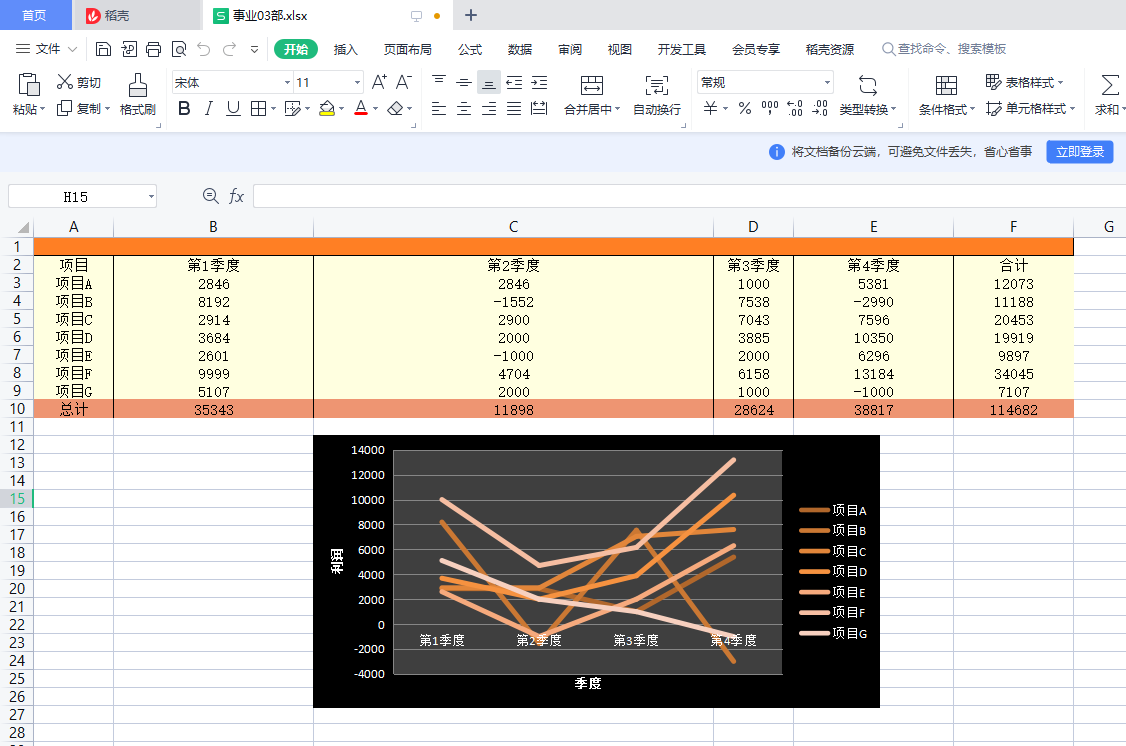## 功能拆解

在分析项目、功能拆解前，先认识一下Excel图的元素。

X、Y轴的标题：图的坐标轴分为X轴（水平轴）、Y轴（垂直轴），其标题说明了坐标轴的内容。如下图所示：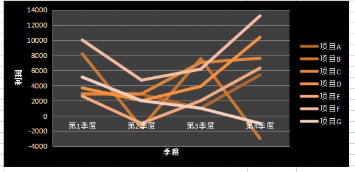类别轴、值轴：坐标轴还可以分为类别轴和值轴。值轴用于描述数量，标签一般是数字；类别轴用于描述类别，标签一般为类别名，如下图的“第n季度”。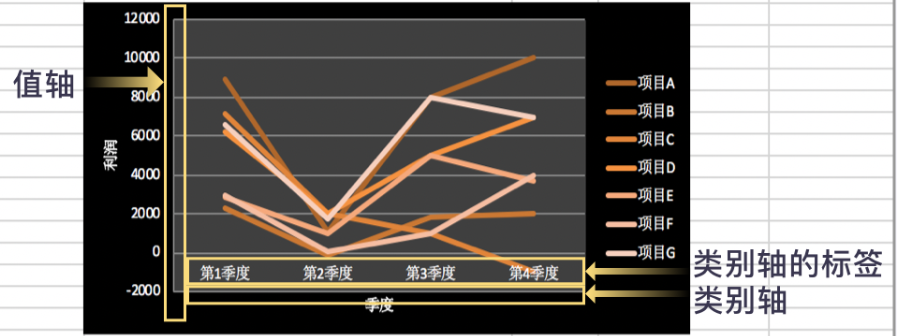图例：说明图中数据系列的含义。在下图中，图例说明相应颜色折线的含义，比如：深褐色折线描述了项目A的利润变化。

简单了解Excel图的元素后，我们开始项目的分析和拆解。

先回顾一下如何使用Microsoft Excel为多个Excel文件绘制折线图。

首先，打开一个Excel文件，绘制利润走势折线图，过程分为两步：
一、折线图绘制
二、折线图信息及样式的优化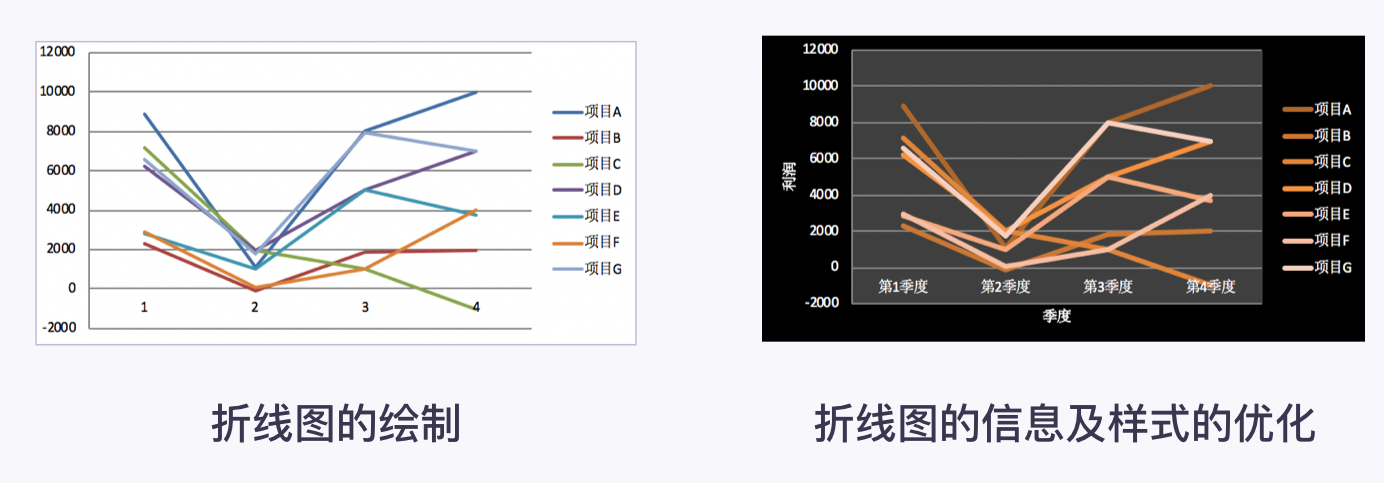其中，折线图绘制包括三个步骤：确认图的类型引用表格的数据设置图在工作表的位置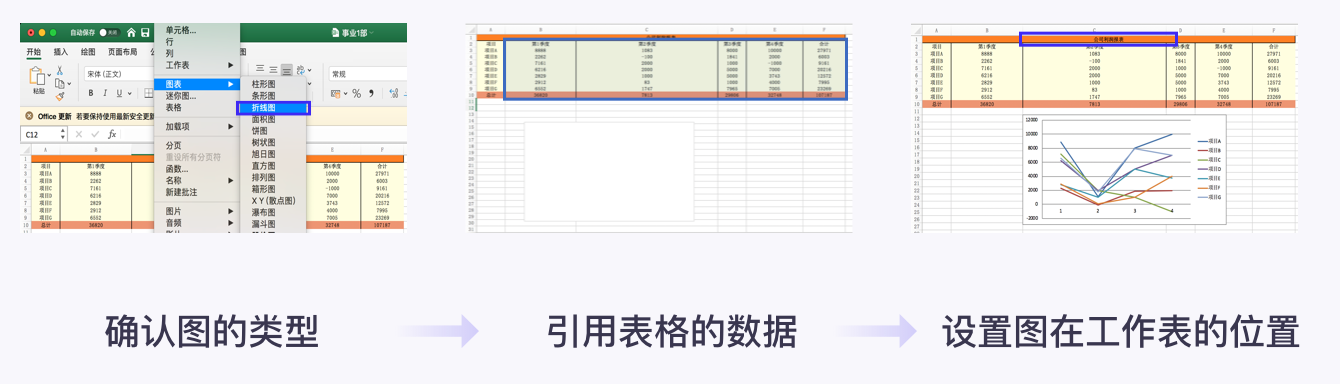折线图信息及样式的优化包括三个步骤：修改类别轴的标签添加X轴、Y轴的标题修改图的样式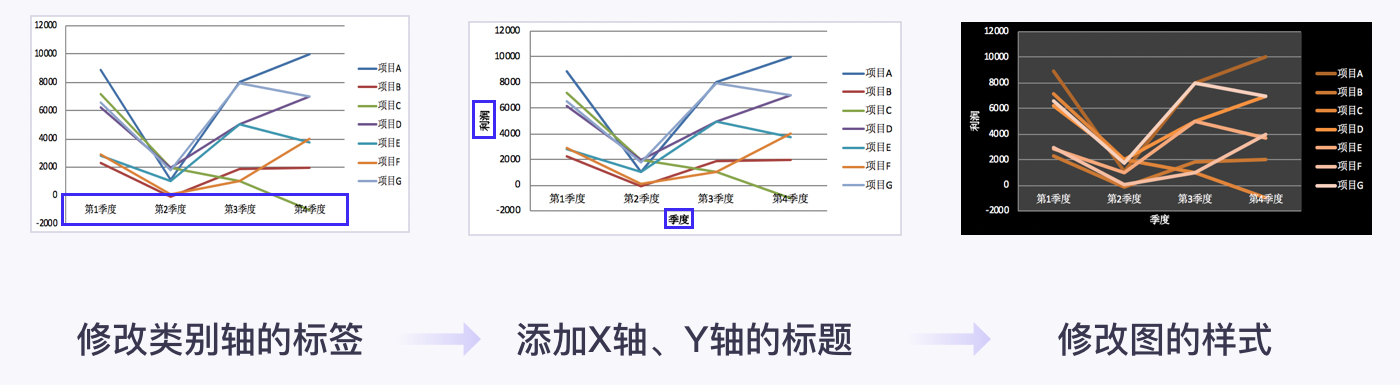最后，依次打开其他Excel文件，重复实现折线图绘制折线图信息及样式的优化

其实，利用Python绘制折线图时，也对应着这两步：折线图绘制折线图信息及样式的优化

由此，该项目可以拆解为四大功能块：循环打开Excel文件折线图绘制折线图信息及样式的优化保存Excel文件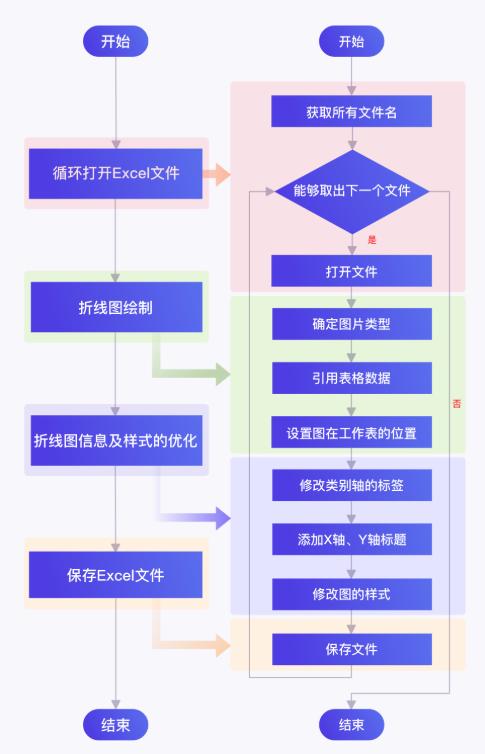项目的关键问题在于：LineChart对象Reference对象的学习，实现折线图绘制折线图信息及样式的优化

然后，结合已学的知识for循环os.listdir()完成循环打开Excel文件工作簿对象.save()完成保存Excel文件

最后，将四个功能块合并，就可以为52个事业部的Excel表格绘制折线图。

# 2. 折线图绘制

折线图绘制功能块包括三个步骤：确定图的类型引用表格中的数据设置图在工作表的位置

接下来，我会讲解各步骤相关的知识点。最后，利用所学知识实现折线图绘制功能块。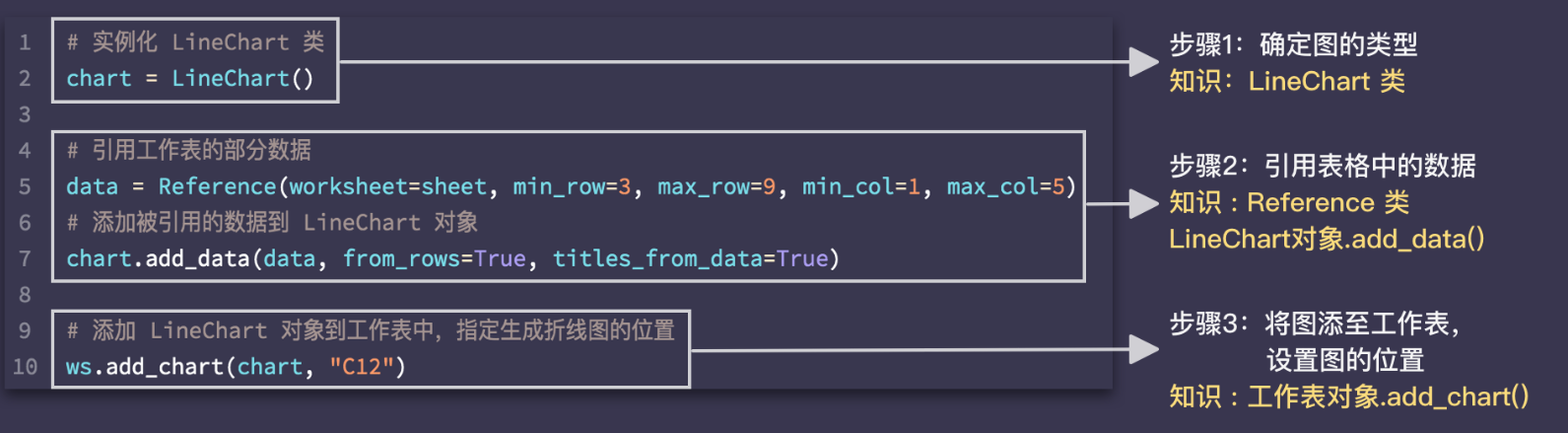## 确定图的类型

### LineChart类

openpyxl 可以绘制条形图、折线图等图。在openpyxl.chart模块中，每个类型的图都有对应的类。

通过chart = LineChart()，得到LineChart对象（LineChart的意思是：折线图)，相当于确认图的类型为折线图。

同理，通过chart = BarChart()可以得到BarChart对象（BarChart的意思是：条形图)。

综上，LineChart对象BarChart对象都可以称为图表对象。

需要注意的是，在实例化LineChart类前，不要忘了从openpyxl.chart中导入LineChart

## 引用表格中的数据

### Reference 类

在Python中，通过实例化openpyxl.chart中的Reference类（Reference中文翻译为：引用），完成表格数据的引用。

例如：Reference(worksheet=ws, min_row=3, max_row=9, min_col=1, max_col=5)引用了工作表对象ws中第3行到第9行、第1列到第5列矩形区域的数据。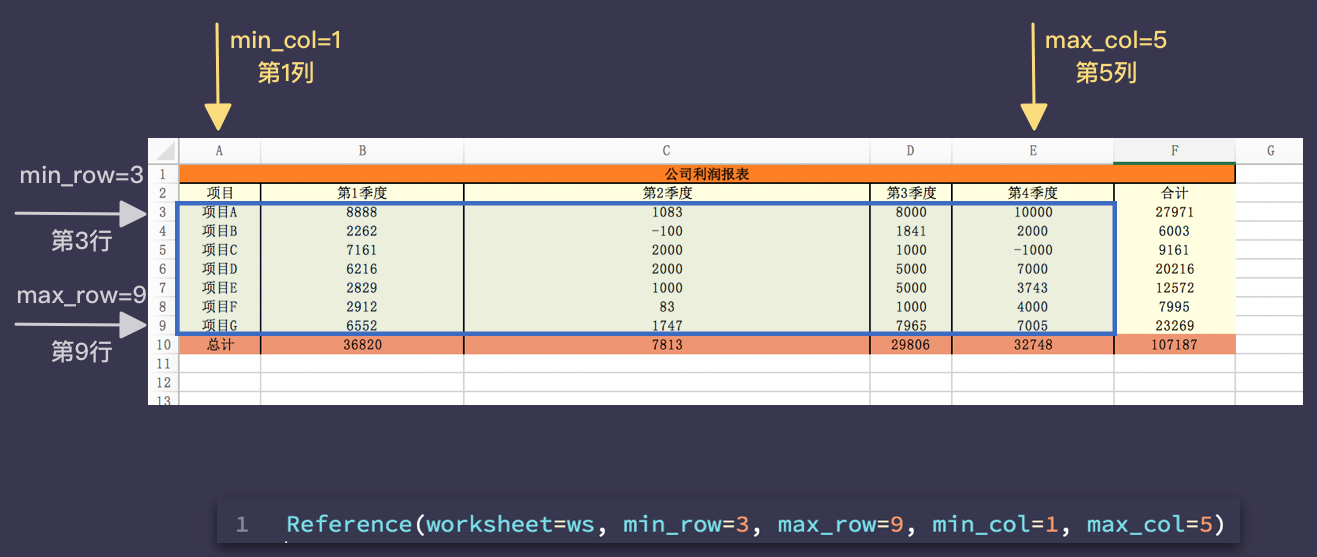Reference 类中的各参数代表什么意思？

参数worksheet比较好理解，指明被引用数据所在的工作表对象。

参数min_rowmax_rowmin_colmax_col：指明被引用数据的区域。

其中，min_row指明数据区域的起始行，max_row、min_col、max_col指明终止行、起始列、终止列。如下图所示。
【温馨提示】区域包括起始行、终止行、起始列、终止列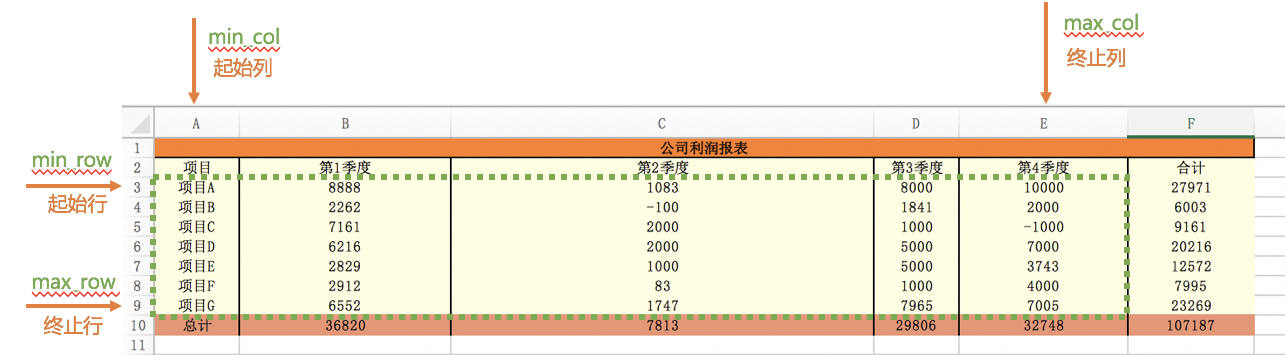通过实例化Reference类，引用“事业01部.xlsx”第 3 行的数据。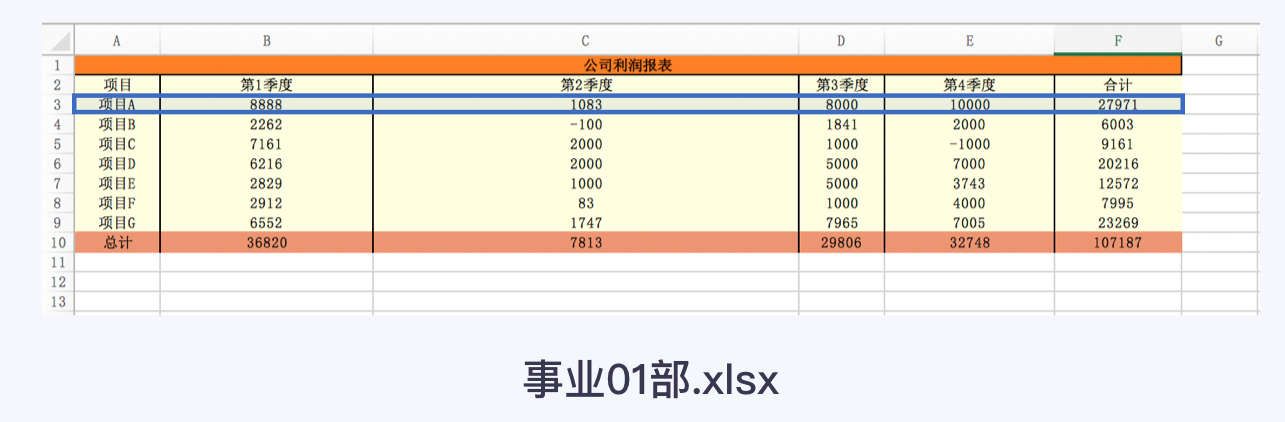实现代码：

from openpyxl import load_workbook
from openpyxl.chart import Reference, LineChart
# 读取工作簿
# 读取工作簿中的活跃工作表
ws = wb.active
# 实例化 LineChart 类
chart = LineChart()
# 实例化Reference类，创建Reference对象，赋值给变量data。引用第3行的数据。
data = Reference(worksheet=ws, min_row=3, max_row=3, min_col=1, max_col=6)

当取一行数据时，起始行和终止行是同一行。

通过实例化Reference类引用表格的数据后，还需要把被引用的数据添加到LineChart对象

这时， LineChart对象.add_data()方法派上用场了。

例如，Linechart对象.add_data(data, from_rows=True, titles_from_data=True)，将被引用的数据data添加到LineChart对象中。

第一个参数是被引用的数据，它是Reference对象

参数from_rows，参数titles_from_data又是什么意思呢？

参数from_rows的值可以是TrueFalse，默认值是False。我们尝试给参数from_rows设置不同的值理解该参数的意义。

数from_rows小结：

一、from_rows（中文翻译为：来自行）。当from_rows设置为True时，引用区域的每行数据绘制一条折线。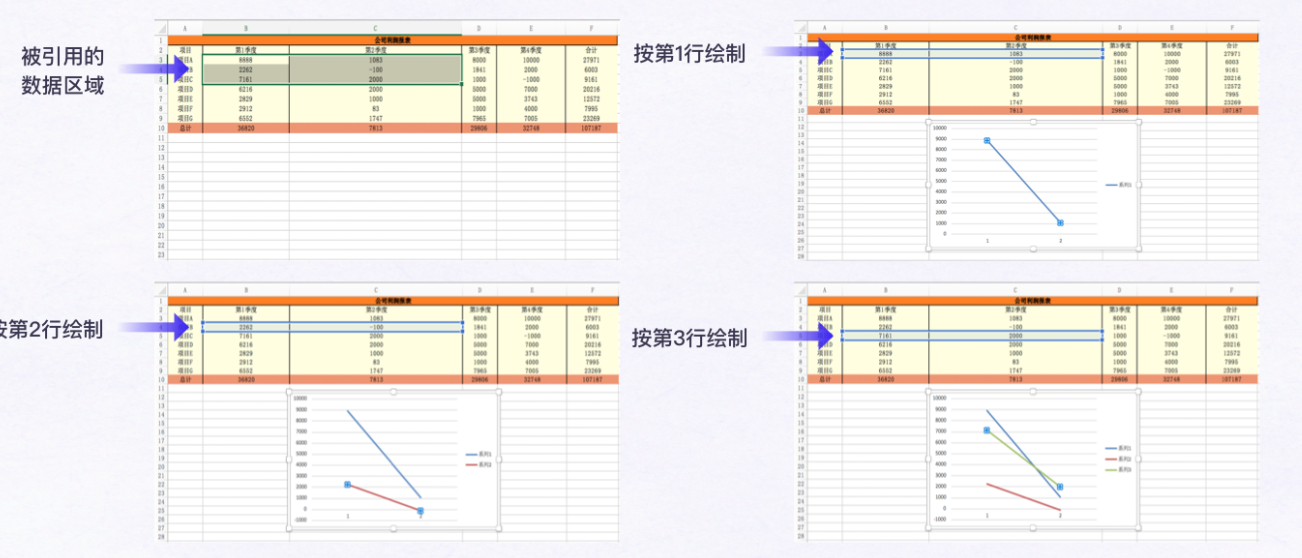二、当from_rows设置为False时，引用区域的每列数据绘制一条折线。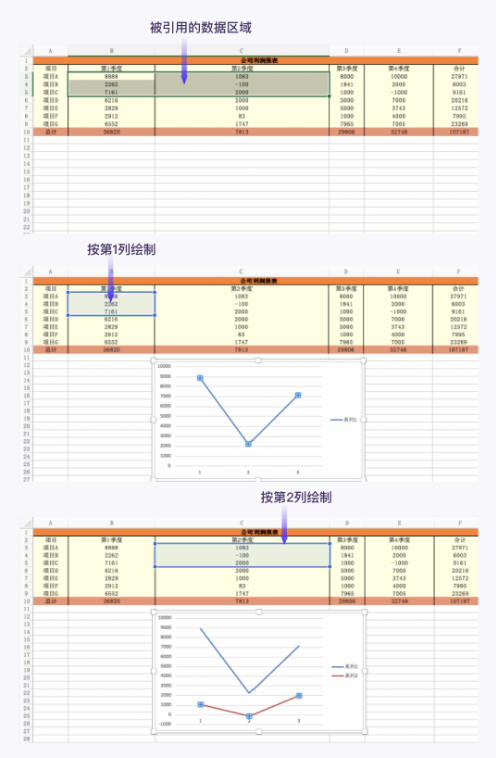参数titles_from_data的值也只能是TrueFalse，默认值是False。当设置不同的值，折线图会是怎么样的呢？

所以，当titles_from_data = False时，只需要引用绘制折线的数据

titles_from_data = True时，则需要多引用表格的数据来命名图例。

如下图中，多引用了表格的A列：项目A、项目B、项目C来命名图例。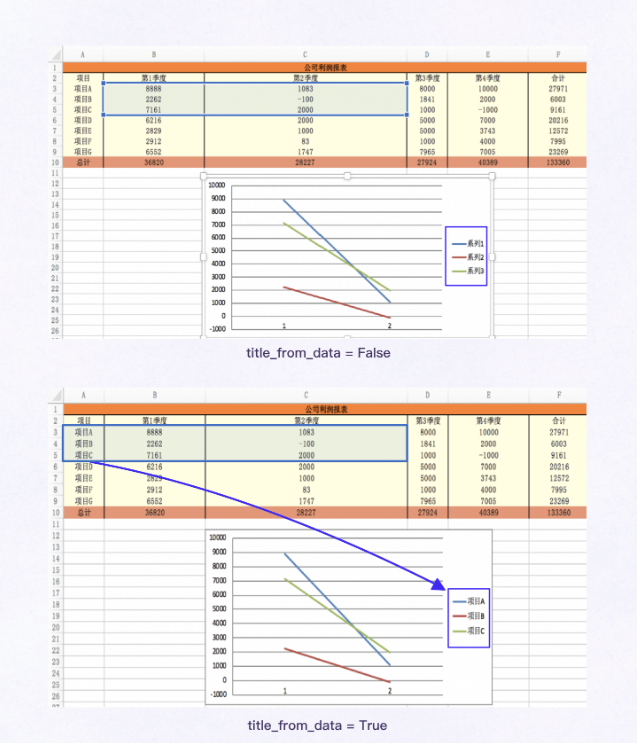引用表格中的数据步骤涉及的知识已讲解完：

1）Reference类：Reference(worksheet, min_row, max_row, min_col, max_col)
2）add_data()方法：LineChart对象.add_data(Reference对象，from_rows, titles_from_data)

了解了如何去引用数据来绘制折线图后，还需要确定好折线图在表格中放置的位置。

## 添图至表格的合适位置

绘制完图后，需要使用方法工作表对象.add_chart()将图添加至工作表，并设置图的位置。

add_chart()的使用很简单：工作表对象.add_chart(图表对象，参数anchor)

第1个参数，指明被添加至工作表的图表对象，这里是LineChart对象。

第2个参数 anchor：设置图在工作表的位置。

例如，ws.add_chart(chart, "C12")，将绘制后的图表对象chart添加到工作表对象ws中，图的左上角位于单元格C12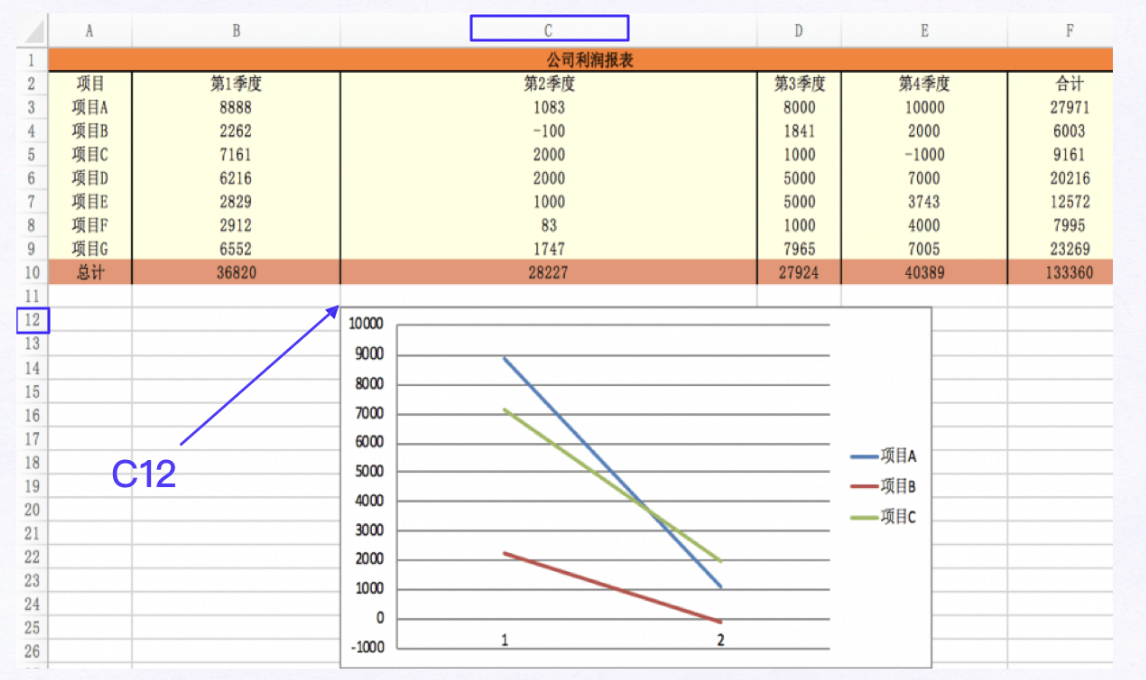至此，我们已经可以在工作表上引用数据绘制出一个折线图，并放置在工作表的固定位置了。也就实现了功能块2：折线图绘制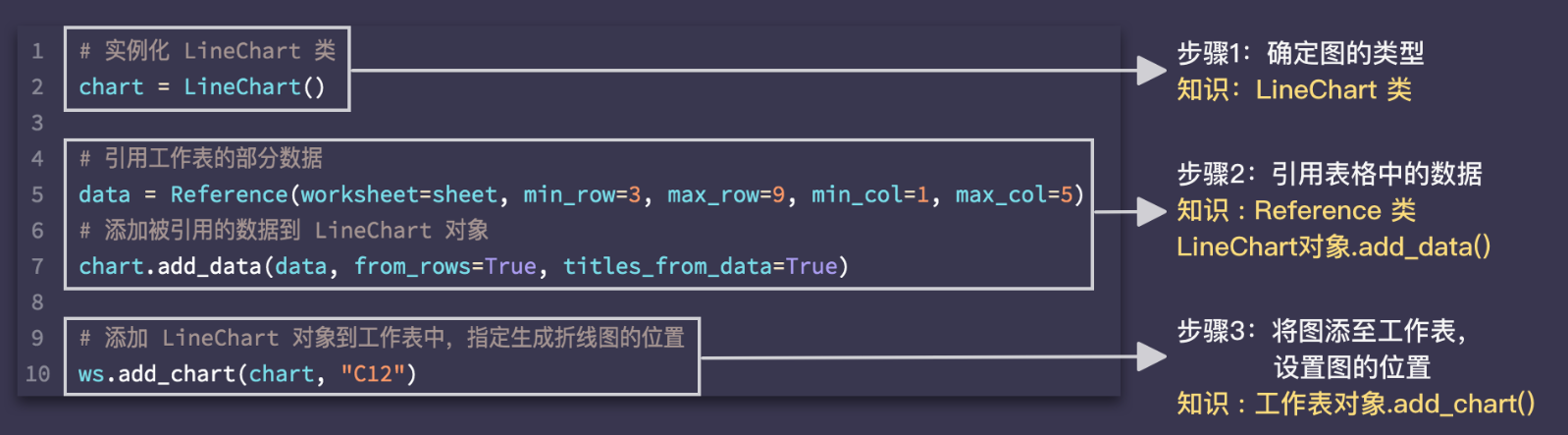练习绘制折线图：

绘制项目A～项目G的利润变化，需要以项目名命名图例。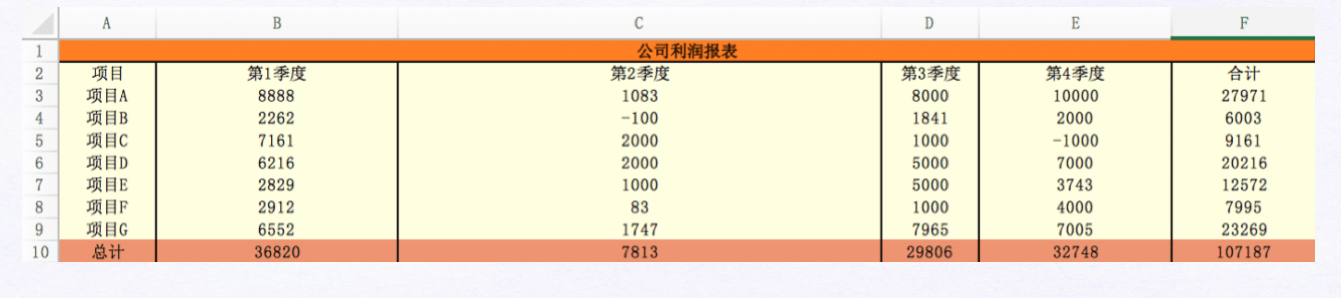涉及到的知识点有：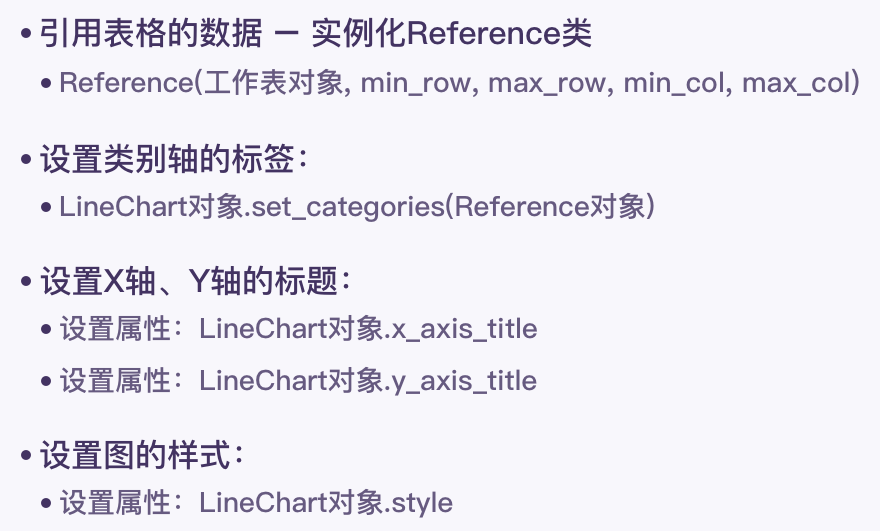实现代码：

from openpyxl import load_workbook
from openpyxl.chart import LineChart, Reference

# 读取工作簿
# 读取工作簿中的活跃工作表
ws = wb.active
# 实例化 LineChart 类，得到 LineChart 对象, 并赋值给变量chart
chart=LineChart()
# 实例化 Reference 类，引用工作表的部分数据。将Reference对象赋值给变量data
data=Reference(worksheet=ws,min_row=3,max_row=9,min_col=1,max_col=5)
# 添加被引用的数据到 LineChart 对象
# 添加 LineChart 对象到工作表中，折线图的左上角位于单元格 C12。

# 保存文件
wb.save('./事业01部.xlsx')


运行效果：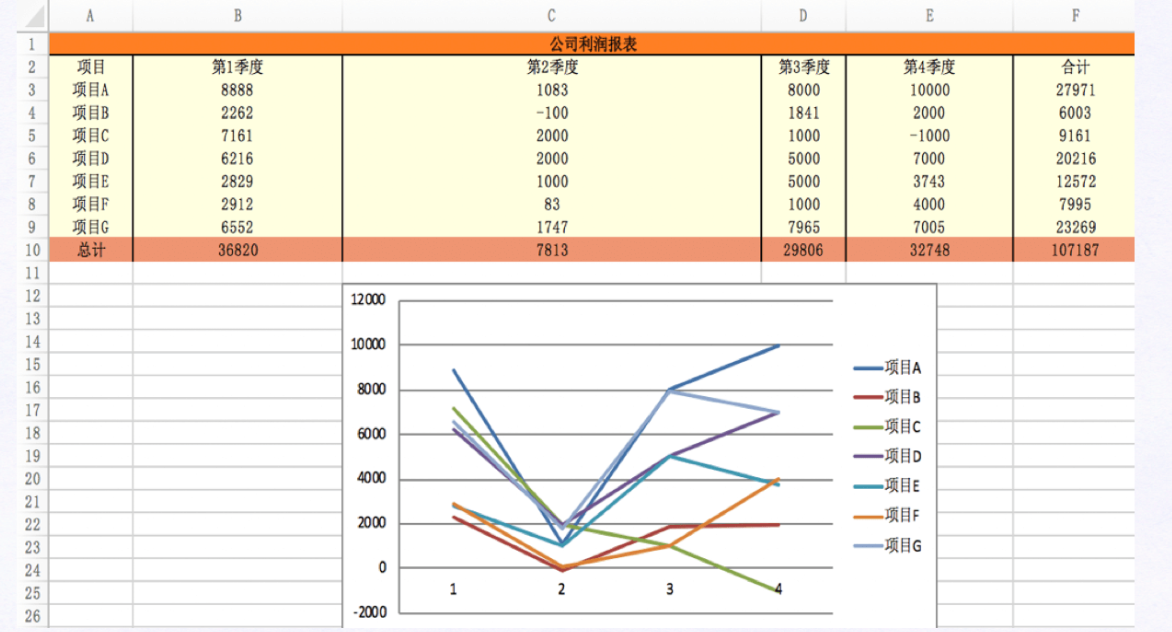展开全文开发语言 后端 Excel
• matplotlib简介matplotlib 是python最著名的绘图库，它提供了一整套和matlab相似的命令API...因此如果你需要绘制某种类型的，只需要在这个页面中浏览/复制/粘贴一下，基本上都能搞定。在Linux下比较著名的数据工...

matplotlib简介

matplotlib 是python最著名的绘图库，它提供了一整套和matlab相似的命令API，十分适合交互式地行制图。而且也可以方便地将它作为绘图控件，嵌入GUI应用程序中。

它的文档相当完备，并且Gallery页面中有上百幅缩略图，打开之后都有源程序。因此如果你需要绘制某种类型的图，只需要在这个页面中浏览/复制/粘贴一下，基本上都能搞定。

在Linux下比较著名的数据图工具还有gnuplot，这个是免费的，Python有一个包可以调用gnuplot，但是语法比较不习惯，而且画图质量不高。

而 Matplotlib则比较强：Matlab的语法、python语言、latex的画图质量（还可以使用内嵌的latex引擎绘制的数学公式）。

绘图库Matplotlib的安装方法：点击这里

matplotlib绘制折线图

1. line chart

import numpy as np

import matplotlib.pyplot as plt

x = np.linspace(0, 2 * np.pi, 100)

y1, y2 = np.sin(x), np.cos(x)

plt.plot(x, y1)

plt.plot(x, y2)

plt.title('line chart')

plt.xlabel('x')

plt.ylabel('y')

plt.show()2. 图例

在plot的时候指定label，然后调用legend方法可以绘制图例。例如：

import numpy as np

import matplotlib.pyplot as plt

x = np.linspace(0, 2 * np.pi, 100)

y1, y2 = np.sin(x), np.cos(x)

plt.plot(x, y1, label='y = sin(x)')

plt.plot(x, y2, label='y = cos(x)')

plt.legend()

plt.show()legend方法可接受一个loc关键字参数来设定图例的位置，可取值为数字或字符串：

0: "best'

1: "upper right'

2: "upper left'

3: "lower left'

4: "lower right'

5: "right'

6: "center left'

7: "center right'

8: "lower center'

9: "upper center'

10: "center'

3. 线的样式

（1）颜色

plot方法的关键字参数color(或c)用来设置线的颜色。可取值为：

1、颜色名称或简写

b: blue

g: green

r: red

c: cyan

m: magenta

y: yellow

k: black

w: white

2、#rrggbb

3、(r, g, b) 或 (r, g, b, a)，其中 r g b a 取均为[0, 1]之间

4、[0, 1]之间的浮点数的字符串形式，表示灰度值。0表示黑色，1表示白色

（2）样式

plot方法的关键字参数linestyle(或ls)用来设置线的样式。可取值为：

-, solid

--, dashed

-., dashdot

:, dotted

'', ' ', None

（3）粗细

设置plot方法的关键字参数linewidth(或lw)可以改变线的粗细，其值为浮点数。

import numpy as np

import matplotlib.pyplot as plt

x = np.linspace(0, 2 * np.pi, 100)

y1, y2 = np.sin(x), np.cos(x)

plt.plot(x, y1, c='r', ls='--', lw=3)

plt.plot(x, y2, c='#526922', ls='-.')

plt.show()4. marker

以下关键字参数可以用来设置marker的样式：

marker

markeredgecolor 或 mec

markeredgewidth 或 mew

markerfacecolor 或 mfc

markerfacecoloralt 或 mfcalt

markersize 或 ms

其中marker可取值为：

'.': point marker

',': pixel marker

'o': circle marker

'v': triangle_down marker

'^': triangle_up marker

'<': triangle_left marker

'>': triangle_right marker

'1': tri_down marker

'2': tri_up marker

'3': tri_left marker

'4': tri_right marker

's': square marker

'p': pentagon marker

'*': star marker

'h': hexagon1 marker

'H': hexagon2 marker

'+': plus marker

'x': x marker

'D': diamond marker

'd': thin_diamond marker

'|': vline marker

'_': hline marker

例如：

import numpy as np

import matplotlib.pyplot as plt

x = np.linspace(0, 2 * np.pi, 10)

y1, y2 = np.sin(x), np.cos(x)

plt.plot(x, y1, marker='o', mec='r', mfc='w')

plt.plot(x, y2, marker='*', ms=10)

plt.show()另外，marker关键字参数可以和color以及linestyle这两个关键字参数合并为一个字符串。例如：

import numpy as np

import matplotlib.pyplot as plt

x = np.linspace(0, 2 * np.pi, 10)

y1, y2 = np.sin(x), np.cos(x)

plt.plot(x, y1, 'ro-')

plt.plot(x, y2, 'g*:', ms=10)

plt.show()总结

以上就是这篇文章的全部内容了，希望本文的内容对大家的学习或者工作能带来一定的帮助，如果有疑问大家可以留言交流，谢谢大家对脚本之家的支持。

展开全文• 本篇文章给大家带来的内容是关于Python绘制折线图和散点图的详细方法介绍（代码示例），有一定的参考价值，有需要的朋友可以参考一下，希望对你有所帮助。1、绘制折线图和散点图要用到matplotlib下的pylab，因此我们...

本篇文章给大家带来的内容是关于Python绘制折线图和散点图的详细方法介绍（代码示例），有一定的参考价值，有需要的朋友可以参考一下，希望对你有所帮助。

1、绘制折线图和散点图要用到matplotlib下的pylab，因此我们需要先引入，因为要用到数组实现，还要引入numpy。然后确定x轴和y轴的数据，最后将其呈现出来。import matplotlib.pylab as pyl

import numpy as np

x = [1, 3, 5, 6, 8, 13, 14, 16]

y = [5, 1, 6, 7, 9, 3, 2, 10]

pyl.plot(x, y)

pyl.show()

这样一个简单的折线图就绘制好了。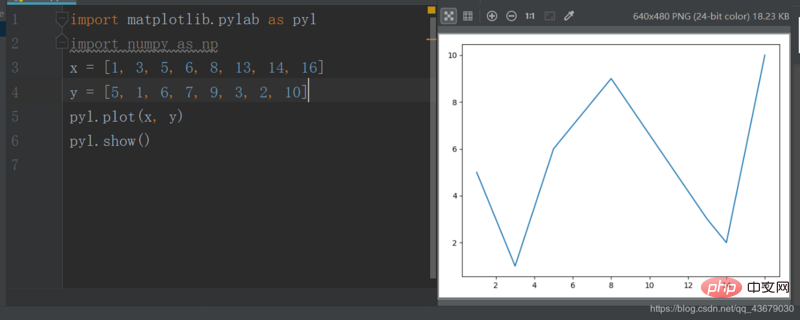上面的plot()有三个参数，第一个参数为x轴坐标，第二个参数为y轴坐标，第三个参数为确定线型，可有可无，如果要将上面的折线图改为散点图，只需更改第三个参数为"o’。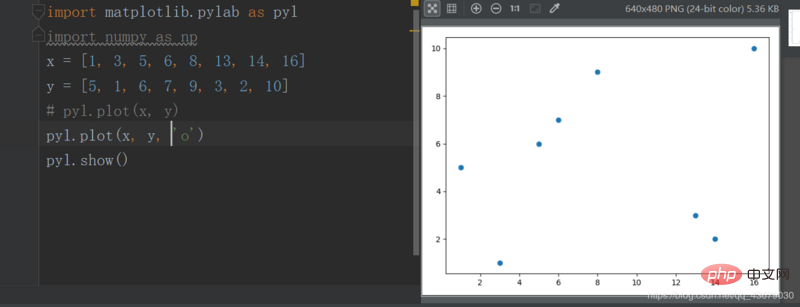如果让折线图和散点图叠加还可以突出每个点。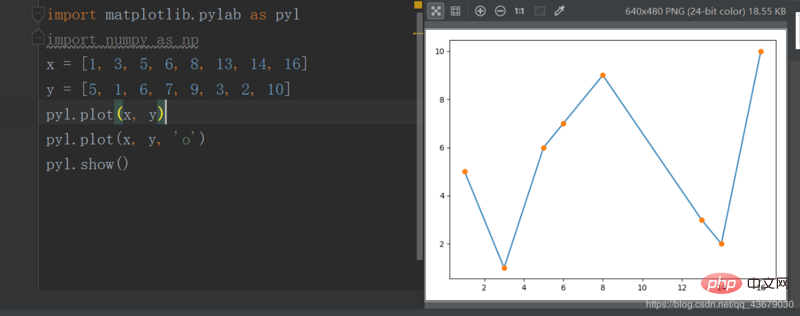2.我们还可以改变线和点的颜色，只需修改plot()的第三个参数。c--cyan--青色

r--red--红色

m--magente--品红

g--green--绿色

b--blue--蓝色

y--yellow--黄色

k--black--黑色

w--white--白色

上述参数可以叠加。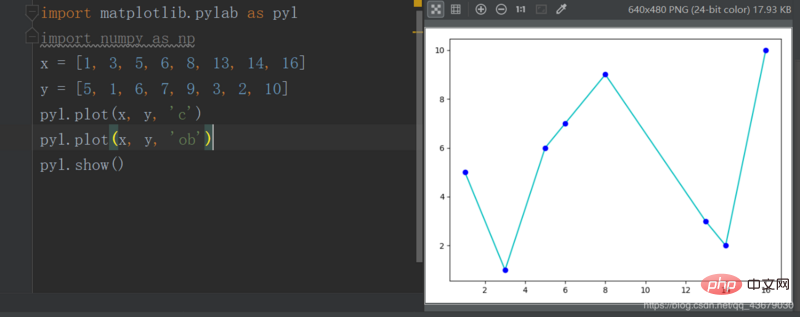3.我们还可以改变线型，也是修改plot的第三个参数。- 实线

-- 虚线

-. 形式即为-.

: 细小的虚线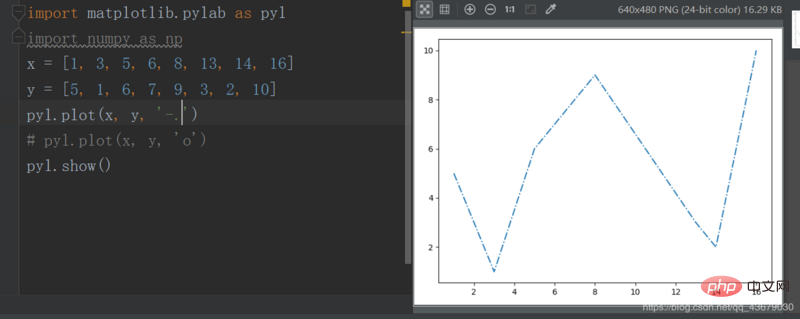4.我们还可以改变点型，同样是修改第三个参数。s--方形

h--六角形

H--六角形

*--*形

+--加号

x--x形

d--菱形

D--菱形

p--五角形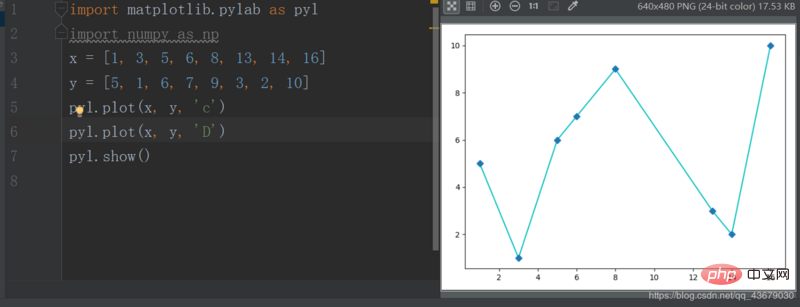5.我们目前绘制的图形，无图像名称及横纵坐标轴的名称，我们需要在程序中添加如下语句pyl.title()

pyl.xlabel()

pyl.ylabel()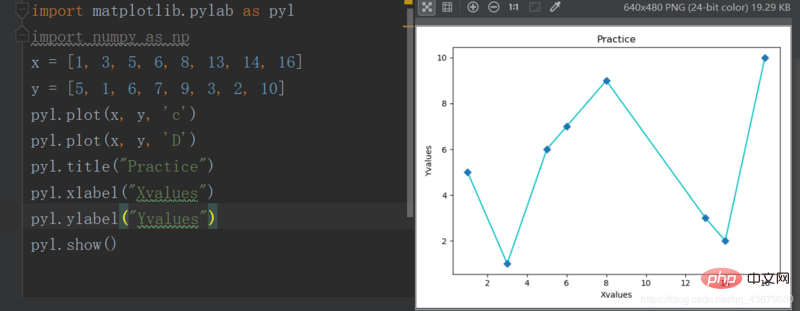6.现在绘图的x,y轴的范围是系统自动生成的，我们要想自定义，需要加上下面的两条语句，括号内为取值范围pyl.xlim()

pyl.ylim()

7.如果要在同一幅图中绘制多个图像，只需在show()之前再定义另外两个变量即可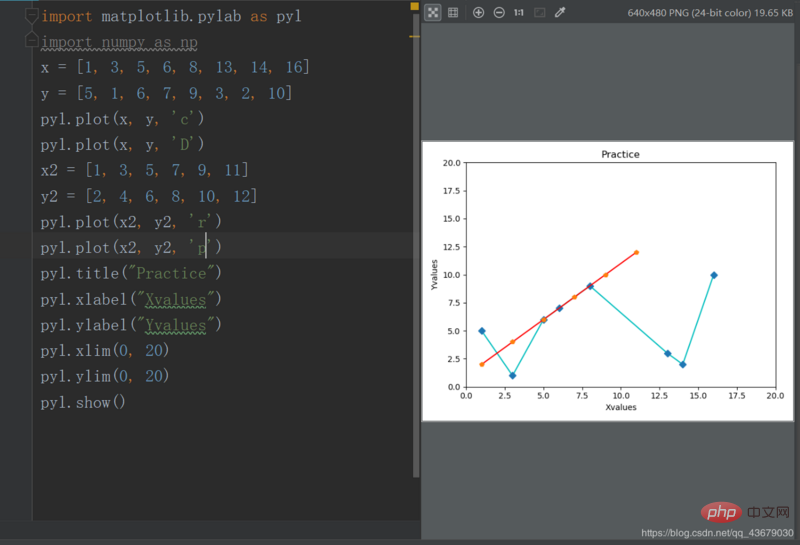以上就是Python绘制折线图和散点图的详细方法介绍（代码示例）的详细内容，更多请关注php中文网其它相关文章！

本文转载于：segmentfault，如有侵犯，请联系a@php.cn删除

展开全文• 最近要论文中的，参考了一下之前的论文里面的，觉得人家的不错（见下），想一下，但是一直也没找到合适的画法，最近潜心研究，终于解决了这个问题，写一下，供大家参考 乍一看，这个挺唬人的，因为它...开发语言
• 前做PPT要用到折线图，嫌弃EXCEL自带的看上去不好看，就用python写了一个画折线图的程序。 import matplotlib.pyplot as plt x=[1,2,3,4,5,6] y1=[35000,85000,120000] y2=[45000,85000,100000] y3=[25000,65000,...
• 使用python绘制折线图过程1、导入库和设置输入折线图数据importnumpyasnpimportmatplotlib.pyplotasplt#x轴刻度标签x_ticks=['a','b','c','d','e','f']#x轴范围(0,1,...,len(x_ticks)-1)x=np.arange(len(x_ticks))#...
• /usr/bin/env python3 # -*- coding: utf-8 -*- import numpy as np from scipy import interpolate import matplotlib.pyplot as plt from matplotlib import rcParams config = { "font.family": 'serif', ...pycharm
• 1.最简单的直线代码如下：import numpy as npimport matplotlib.pyplot as pltx=[0,1]y=[0,1]plt.figure()plt.plot(x,y)plt.savefig("easyplot.jpg")结果如下：代码解释：#x轴，y轴x=[0,1]y=[0,1]#...
• matplotlib是python中用于数据可视化最常用的包，如果系统中未安装matplotlib包，可以在命令行使用pip install matplotlib安装matplotlib包下面我们开始使用matplotlib....[1, 4, 9, 16, 25]#绘制折线图并设置线条宽...
• matplotlib是Python中的一个第三方库。主要用于开发2D图表，以渐进式、交互式的方式实现数据可视化，可以更直观的呈现数据，使数据更具说服力。 一、安装matplotlib pip install matplotlib -i ...
• python画折线图利用的是matplotlib.pyplot.plot的工具来绘制折线图，这里先给出一个段代码和结果图：# -*- coding: UTF-8 -*-import numpy as npimport matplotlib as mplimport matplotlib.pyplot as plt#这里导入...
• python画折线图参数配置 1.设定x，y坐标轴间隔 2.中文显示,搞定 3.网格线显示，搞定 4.数值显示，简化阅读繁琐程度 5.设置折线的颜色 #解决中文显示问题 plt.rcParams['font.sans-serif']=['SimHei'] plt....
• 利用Python绘制科研论文折线图，通过读取exal大量数据，进行折线图绘制。机器学习
• 主要介绍了Python 绘制可视化折线图的方法，文中讲解非常细致，代码帮助大家更好的理解和学习，感兴趣的朋友可以了解下
• ## Python绘制折线图、散点图

万次阅读 多人点赞 2019-01-06 11:01:13
Python绘图需要下载安装matplotlib模块，它是一个数学绘图库，我们将使用它来制作简单的图表，如折线图和散点图。关于matplotlib模块的下载安装预配置将在后面具体介绍。 （一）绘制折线图 首先，我们先绘制一个...大数据 机器学习
• 今天教大家用python绘制一些线性图案，需要的朋友可以借鉴参考一下。最简单的直线代码如下：import numpy as npimport matplotlib.pyplot as pltx=[0,1]y=[0,1]plt.figure()plt.plot(x,y)plt.savefig("...
• 这篇文章利用的是matplotlib.pyplot.plot的工具来绘制折线图，这里先给出一个段代码和结果图： # -*- coding: UTF-8 -*- import numpy as np import matplotlib as mpl import matplotlib.pyplot as plt #这里导入...
• 折线图绘制主要分为四步： 1）导入相关的库 2）做图片输出的通用设置 3）设置数据及折现的属性 4）设置图的标题 案例： import matplotlib import numpy as np from matplotlib import pyplot as plt %...matplotlib
• 首先安装plt相关的包，其实也就是画图相关的包，好像叫做Matplotlib 无所谓，安装包的命令行给你，直接粘，然后复制代码，直接跑就好 pip install pytest-plt 下面就是代码了 import matplotlib.pyplot as plt ...
• 添加如下代码即可： import matplotlib matplotlib.rcParams['axes.unicode_minus']=False
• 完成上面的折线图绘制后，目前工作表的折线图应该是长这样的： 这折线图存在一些问题：信息模糊；不清楚X轴、Y轴描述了什么数据；折线图的样式不是很美观。 所以，还需要进行折线图信息及样式的优化，具体可...开发语言 后端 excel
• 构建python文件，使用import函数引入两个类，使我们可以绘制图像并对图像样式进行改变使其更加明显美观。 值得一提的是，matplotlib绘制出的图像会出现无法显示中文的情况，这并不是因为它不具备中文字体，而是并...
• 今天教大家用python绘制一些线性图案，需要的朋友可以借鉴参考一下。最简单的直线代码如下：import numpy as npimport matplotlib.pyplot as pltx=[0,1]y=[0,1]plt.figure()plt.plot(x,y)plt.savefig("easyplot....
• ## 利用python画折线图

千次阅读 2020-12-04 15:26:12
mfc='w',label=u'y=x^2曲线') plt.plot(x, y1, marker='*', ms=10,label=u'y=x^3曲线') plt.legend() # 让图例生效 plt.xticks(x, names, rotation=45) plt.margins(0) plt.subplots_adjust(bottom=0.15) plt.......